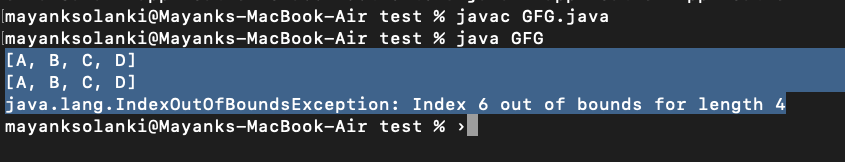GeeksforGeeks App
Open AppBrowser
Continue

# How to Replace a Element in Java ArrayList?

To replace an element in Java ArrayList, set() method of java.util. An ArrayList class can be used. The set() method takes two parameters-the indexes of the element which has to be replaced and the new element. The index of an ArrayList is zero-based. So, to replace the first element, 0 should be the index passed as a parameter.

Declaration:

`public Object set(int index, Object element)`

Return Value: The element which is at the specified index

Exception Throws: IndexOutOfBoundsException
This occurs when the index is out of range.

`index < 0 or index >= size()`

Implementation:

Here we will be proposing out 2 examples wherein one of them we will be setting the index within bound and in the other, we will be setting the index out of bounds.

Example 1: Where Index is Within Bound

## Java

 `// Java program to demonstrate set() Method of ArrayList``// Where Index is Within Bound` `// Importing required classes``import` `java.io.*;``import` `java.util.*;` `// Main class``class` `GFG {` `  ``// Main driver method``  ``public` `static` `void` `main(String[] args) {` `    ``// Try block to check for exceptions``    ``try` `{` `      ``// Creating an object of Arraylist class``      ``ArrayList list = ``new` `ArrayList<>();`  `      ``// Adding elements to the List``      ``// using add() method` `      ``// Custom input elements``      ``list.add(``"A"``);``      ``list.add(``"B"``);``      ``list.add(``"C"``);``      ``list.add(``"D"``);` `      ``// Print all the elements added in the above object``      ``System.out.println(list);` `      ``// 2 is the index of the element "C".``      ``//"C" will be replaced by "E"``      ``list.set(``2``, ``"E"``);` `      ``// Printing the newly updated List``      ``System.out.println(list);` `    ``}` `    ``// Catch block to handle the exceptions``    ``catch` `(Exception e) {` `      ``// Display the exception on the console``      ``System.out.println(e);``    ``}``  ``}``}`

Output

```[A, B, C, D]
[A, B, E, D]
```

Example 2: Where Index is Out of Bound

## Java

 `// Java program to demonstrate set() Method of ArrayList``// Where Index is Out of Bound` `// Importing required classes``import` `java.io.*;``import` `java.util.*;` `// Main class``class` `GFG {` `  ``// Main driver method``  ``public` `static` `void` `main(String[] args) {` `    ``// Try block to check for exceptions``    ``try` `{` `      ``// Creating an object of Arraylist class``      ``ArrayList list = ``new` `ArrayList<>();`  `      ``// Adding elements to the List``      ``// using add() method` `      ``// Custom input elements``      ``list.add(``"A"``);``      ``list.add(``"B"``);å``      ``list.add(``"C"``);``      ``list.add(``"D"``);` `      ``// Print all the elements added in the above object``      ``System.out.println(list);` `      ` `      ``// Settijg the element at the 6 th index which``      ``// does not exist in our input list object``      ``list.set(``6``);``  ` `      ``// Printing the newly updated List``      ``System.out.println(list);``    ``}` `    ``// Catch block to handle the exceptions``    ``catch` `(Exception e) {` `      ``// Display the exception on the console``      ``System.out.println(e);``    ``}``  ``}``}`

Output:My Personal Notes arrow_drop_up